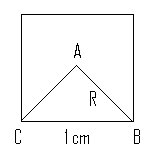## Oct 16, 2006

### Calculating the value of pi

I accept that this post looks a bit out of context for SQL, but its defenitely an interesting "garbage" to collect. For all having some aptitude in maths, you would wonder how the value of pi is derieved? Here is a small attempt in finding the value of pi from what we already know.

This is not a new method for which I can take ownership. Its a widely known method which I am just putting here.

Lets take the simple formula for the circumference of the circle.

S = 2*pi*R

which means that
pi = S/2R

Now S is the circumference of the circle. So if we know the radius and circumference of a circle accurately we can get the value of pi.

Now, getting that is a bit tough, so we will have to go by approximation.

For example, lets take a square (polygon with 4 sides) of side 1 cm in length. Now, I can call a sqaure as an approximate circle (very approximate indeed).
Now the radius of this square is approxmately half the length of the diagonal (or the length of one of the line of the angle foming the vertex. Lets call it R.
Take a look at this figure.Say, the number of sides in this square is N (4 in this case)

Perimeter (S) of the square is also N (since the side of the square is 1)
angle A = 360/N degrees = 360/4 = 90 degrees

AB = AC = R (or radius)
angle B = angle C = (180 - (360/N))/2 = 90/2 = 45

By the sine law, we have
Sin(C)/R = Sin(A)/1 cm
=> R = Sin(C)/Sin(A)
=> R = Sin(45)/Sin(90)
=> R = 0.7071

From the formula,
pi = S/2R = 4/(2*0.7071) ~ 2.828

Now this is the approximate value of pi we get when we considered the square to be a circle.
Now as we increase the number of sides in the polygon, the shape gets closer to a circle.
By definition, a circle is a polygon with infinite sides.

Here is where we get in SQL Server to see how this theory hold good as we increase the number of sides. Please note that the query given below is to test the above theory and is not proposed as a way to calculate pi in SQL Server.

The following query gives the corresponding value of pi as we increase the number of sides (in steps of 2) from 4 to 65532. Run the query and check out the result for yourself.

declare @seed int
set @seed = 4
-- The step decides how many sides you want to add to the polygon for each iteration
declare @steps int
set @steps = 2;
with cte as
(select @seed as no_of_sides_in_the_polygon ,
cast(360.0/(@seed) as decimal(38,35)) vertex_angle,
union all
select no_of_sides_in_the_polygon + @steps,cast(360.0/(no_of_sides_in_the_polygon + @steps) as decimal(38,35)),
sin(pi()/180*(180.0 - 360/(no_of_sides_in_the_polygon + @steps))/2)/sin(pi()*2/(no_of_sides_in_the_polygon + @steps))
from cte
where (no_of_sides_in_the_polygon - @seed + @steps)/@steps <32765)
select cte.*,
pi() as PI_From_SQL_Server
from
cte option (maxrecursion 32765)

Try changing the step to 2000 with the seed as 4 and you will notice that, when the number of sides of the polygon reaches 50 million (in the result), you will have corresponding the value of pi equal to the result you get from this query.

select pi()

The above query was written for SQL Server 2005 (using cte). It can be rewritten by using number tables if you get the idea.
Try this query. It gives the value of pi when you aprroximate a 100 million side polygon to a circle.

declare @No_Of_Sides int
set @No_Of_Sides = 100000000

select pi() as given_value_of_pi, cast(0.5*@No_Of_Sides/(sin(pi()/180*(180.0 - 360/(@No_Of_Sides))/2)/sin(pi()*2/(@No_Of_Sides))) as decimal(38,36)) as computed_val_of_pi

if you are looking at calculating the value of Pi without using any of the built-in functions, then you may want to use this method. And its just an implementation of another well known method.

set nocount on

declare @iteration bigint
set @iteration = 100000 /* increase the iteration to get more precision */

create table #values
(
id bigint identity(0,1) primary key,
divisor as id*2 + 1,
multiplier as (id*2 + 2)%4 - 1
)

declare @count bigint
set @count = 0
while (@count<= @iteration)
begin
insert into #values default values
set @count = @count + 1
end

/*This query shows how the value of pi converges to the actual value as the iteration increases */
select  a.id + 1 as iteration,
sum(cast (4.0 as decimal(38,35))* b.multiplier/b.divisor)
from
#values a,
#values b
where
b.id < =a.id
group by
a.id

drop table #values

Anonymous said...

cool!

Anonymous said...